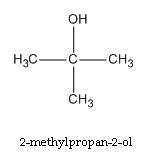## Isomerism of Alcohol

Subject: Chemistry

#### Overview

Alcohols exhibits following three types of isomerism. They are Chain isomerism.Position isomerism. Functional isomerism.In the common system of nomenclature,alcohols are named as. $$Alkyl\,+alcohol=Alkyl\,alcohol$$ Structural formula of tertiary butanol is given below and its IUPAC name is 2.methyl propane-2ol

### Isomerism of alcohols.

Alcohols exhibits following three types of isomerism.

• Chain isomerism.
• Position isomerism.
• Functional isomerism.

Chain isomerism.

Alcohols containing at least 4-carbon atoms form chain isomerism due to the different structure of C-skeleton in the longest chain.

Position isomerism.

Alcohols containing at least 3 C atoms form position isomerism due to a different position of a hydroxy group (OH).

Example.Functional isomer.

Alcohols containing at least 2 carbon atoms give functional isomers. The functional isomer of an alcohol is ether.

Example.

#### Nomenclature.

1. Common system.

In common system of nomenclature,alcohols are named as.

$$Alkyl\,+alcohol=Alkyl\,alcohol$$

Example.

$$alkane\xrightarrow{-ane\,+yl}alkyl\xrightarrow{alcohol}Alkyl\,alcohol$$

$$\underbrace{CH_4}_{Methane}\xrightarrow{-H}\underbrace{CH_3}_{Methyl}-\xrightarrow{+OH}\underbrace{CH_3OH}_{Methyl\,alcohol}$$2. IUPAC systems.

In this system, alcohols are given their respective names by adding suffix-'ol' with word root.

$$Alkane\xrightarrow{+ol}Alkanol$$

Example.

$$CH_4\xrightarrow{+Ol\,-H}\underbrace{CH_3OH}_{Methanol}$$.vWrite down the structural formula and IUPAC name of tert.Butyl alcohol.

Structural formula of tertiary butanol is given below and its IUPAC name is 2.methyl propane-2olSome more example of AlcoholReference.

Bahl, B S, Bahl, and Arun. Advanced Organic chemistry. S. Chand and company Ltd., n.d.

Sthapit, M K, R R Pradhananga, and K B Bajracharya. Foundations of chemistry. Taleju Prakashan, n.d.

Tewari, K S, S N Mehrotra, and N K Vishnoi. A textbook of organic chemistry. Vikash publishing House Pvt. ltd., n.d.

Verma, N K and S K Khanna. Compressive chemistry. 8th edition. Laxmi publications P. Ltd., 1999.

##### Things to remember
1. Alcohols exhibits following three types of isomerism.
2. Alcohols containing at least 4-carbon atoms form chain isomerism due to the different structure of C-skeleton in the longest chain.
3. In the common system of nomenclature,alcohols are named as.

4. $$Alkyl\,+alcohol=Alkyl\,alcohol$$

5.

• It includes every relationship which established among the people.
• There can be more than one community in a society. Community smaller than society.
• It is a network of social relationships which cannot see or touched.
• common interests and common objectives are not necessary for society.